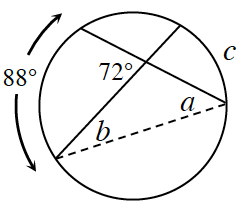### Home > INT2 > Chapter 11 > Lesson 11.2.1 > Problem11-57

11-57.In problem 11-33, you used the relationship between the segment lengths formed by intersecting chords to calculate a missing length. But how are the measures of arcs intercepted by two intersecting chords related? Examine the diagram at right.

1. Solve for $a, b,$ and $c$ using what you know about inscribed angles and the sum of the angles of a triangle.

$2a = 88°$ and $2b = c$

$a = 44°$, $b = 28°$, and $c = 56°$

2. Compare the result for $c$ with $88°$ and $72°$. Is there a relationship?

Find the average of the two arc lengths.